﻿ The Universe as a Quantum Leap, the Schrödinger Equation Links Quantum Mechanics to General RelativityPublications are Open
Access in this journal
Article Versions
Export Article
• Normal Style
• MLA Style
• APA Style
• Chicago Style
Research Article
Open Access Peer-reviewed

### The Universe as a Quantum Leap, the Schrödinger Equation Links Quantum Mechanics to General Relativity

Jarl-Thure Eriksson
International Journal of Physics. 2020, 8(2), 64-70. DOI: 10.12691/ijp-8-2-4
Received May 27, 2020; Revised June 28, 2020; Accepted July 07, 2020

### Abstract

Big Bang, dark energy and dark matter are the main ingredients of the governing paradigm of astrophysics today. The scenario, however, faces several substantial question marks, such as the cause of the Big Bang singularity, the sudden inflationary expansion and the consistence of dark matter. There are also divergent theories about the origin of dark energy. A new theory, CBU standing for the Continuously Breeding Universe, has been developed in order to find solutions based on known principles of physics. The theory incorporates important ideas from the past. The universe is considered as a complex emerging system, which starts from the single fluctuation of a positron-electron pair. Expansion is driven by the appearance of new pairs, which “stay alive” due to a Planck time far larger than the period between fluctuations. It is shown that the gravitational potential energy is the negative counterpart that balances the increase of energy due to new matter. The gravitational parameter G (Newton’s gravitational constant) is inversely proportional to the Einsteinian curvature radius r. As a result the Planck length and Planck time tP are dependent of the curvature and hence by the size of the universe. Here we show that the solution to the Schrödinger equation of an initial positron-electron fluctuation includes an exponential function parameter equal to the Planck length as determined by definition. This gives a strong argument in favour of the CBU theory. Further, the existence of a wave function of the initial event provides a link between quantum mechanics and the theory of general relativity. The universe is a macroscopic manifestation of the quantum world.

### 1. Introduction

In 1948 Fred Hoyle, 1, and separately Hermann Bondi & Thomas Gold, 2, proposed a steady state model, which would maintain expansion but keep the density constant by creating new matter. E. P. Tryon, 3 suggested in 1973 that the universe was initiated by a positron-electron quantum fluctuation. The renown British physicist Paul Dirac wrote in 1974: "One might assume that nucleons are created uniformly throughout space, and thus mainly in intergalactic space. We may call this additive creation”, 4. Alan Guth has hypothesized that the total energy of the universe is zero, 5. Matter and radiation provide the positive part, while the potential energy of gravity forms the negative part. This is a logical description of the connection between space, gravitation and matter.

In three published papers the author has analysed the consequences of postulating an initial event due to a positron-electron fluctuation and a continuous inflow of new matter. The main theory was outlined in Ref. 6, wherein the Coriolis effect was analysed and arguably found responsible for the rotational patterns of the galaxies. The theory was entitled the Continuously Breeding Universe, CBU. In Ref. 7 dark energy was explained as the “free” kinetic energy of incoming matter during the different stages of expansion. Starting from the momentum change the equation for an equivalent cosmological constant was derived. The result led to a consistent solution of the Friedmann-Robertson-Walker kinetic energy equation. The third article, Ref. 8, deals with the influence of an age dependent gravitational parameter. If the parameter G was essentially stronger during time passed, then it would have an impact on the cosmological redshift and thereby change the time scaling of celestial events.

According to the CBU theory the energy is confined to matter and radiation, neither dark energy nor dark matter is required.

### 2. Hypothesis

In principle the universe must be considered as a black hole, because light is confined to the space of the universe, there is no space on the outside. Bernard McBryan has studied black holes of different modes, 9. He states that one could live in a low-density black hole without knowing it. According to his classification our universe could be a “classical finite height black hole”, wherein the ‘photon sphere radius’ is half the Schwarzschild radius. We make this a fundamental law by utilizing the Schwarzschild horizon equation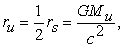(1)

Where ru is the overall radius of the universe (2 times r, the radius of the observable universe), G is the gravitational parameter (Newton’s gravitational constant), Mu = Wu/c2 is the total mass, Wu is the energy of the universe.

In 1917 Albert Einstein, 10, concluded that the curvature radius equals a value 4/3 times that of eq. (1). In 1953 Dennis Sciama, 11 (mentor of Stephen Hawking) arrived at the same equation when trying to explain Mach’s principle, which says that the total mass of the universe is the cause of inertia. Brans and Dicke, 12, also suggested the same relation between radius and energy in 1961. Recently (2015) Fahr and Sokaliwska have developed a model, which supports the validity of eq. (1), 13.

The reason why eq. (1) still applies even if the universe is considered as a low-density black hole, is the fact that a considerable part of the mass energy is confined in the black holes of the galaxy centers thus maintaining an average density in accordance with eq. (1).

### 3. The Birth of the Universe

The initial event created by the occurrence of a positron-electron pair is schematically described in Figure 1. The radius ri is the radius of the “observable universe” of each single particle, but also the curvature radius of the connecting arc between the charges. We write the energy equation of the system as follows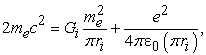(2)

where me and e are the electron mass and charge respectively and ε0 is the permittivity of vacuum.

• Figure 1. The initial event, according to the hypothesis the universe started from a positron-electron fluctuation

Given that Mu = 2 me and ru = 2ri we have from eq. (1)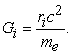(3)

We can now solve ri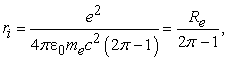(4)

where Re is the classical electron radius. We have ri = 0,533379·10-15 m.

By denoting the two terms on the righthand side of eq. (2) WG and WE, we can define the Eddington version of the Dirac Large Numbers Hypothesis (LNH): ND = (WG+WE)/WG. For the present universe the number is 4,171·1042. At the initial event the number appears to be 2π, a salute to Paul Dirac who introduced the reduced Planck constant h/2π.

Next we want to determine the connection between the radius r and the energy content of the universe. We postulate that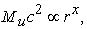(5)

where x is a still unknown exponent. In order to determine x we put the ratio W/rx at the initial event equal to that of the present state, wherein r0 is the radius of the observable universe today. The analogy equation takes the form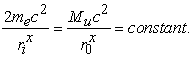(6)

From the latest Planck 2018 results, 14, we have Ωb = 0,049, the baryonic parameter, ρcr = 8,54·10-27 kg/m3, critical density, r0 = 4,394·1026 m, radius of the observable universe. The mass of the observable universe is Mobs = 1,487·1053 kg. The total mass is 8 times larger. x is solved from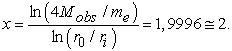(7)

In spite of some inaccuracy in both Mobs and r0 the result clearly shows that the positive energy of the universe is proportional to the radius squared. The result emphasizes of the validity of eq. (2).

We are now able to write the equation for the universe energy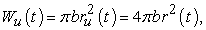(8)

where b is a universal constant, the definition involving 4π was made by the present author. We use the initial event to obtain the constant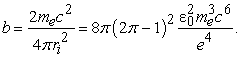(9)

We have b = 0,4580139·1017 J/m2.

Equation (8) can be seen as an analogy to the Bekenstein-Hawking black hole entropy, which correlates with the surface of the event horizon, i.e. rs2.

Rewriting eq. (1) we can express the gravitational parameter G as a function of r (the radius of the observable universe)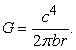(10)

G is a measure of the curvature of space. It is a function depending on the location, close to a mass concentration m, say, G increases. The increase is however so small that it has almost no practical significance on G itself: ∆G/G ≈ m/Mu, e.g. mMilkyWay ≈ 2,3·1041 kg, ∆G = 1,9·10-13 G.

The present radius of the observable universe, r0, is obtained from eq. (10) by assuming GN = 6,67430·10-11 m3/(kgs2). We have r0 = 4,205508·1026 m, slightly smaller than the official estimate of 4,394·1026 m.

### 4. The Expanding Universe

Albert Einstein used the Eulerian hydrodynamical equations for an incompressible fluid to describe the dynamic equations of general relativity. He also introduced the concepts of the density ρ and the pressure p into his theory 15.

The following text is a summary of the derivation worked out by the author in Refs 6 and 7. According to the Friedmann-Robertson-Walker metric the acceleration equation is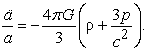(11)

The pressure p represents the driving mechanism for the expansion. Both mass and radiation energy are included in the density ρ.

We introduce the scale factor a = r/r0. By differentiating the energy relation of eq. (8) we have dW = 8πbr·dr = -pdV. Then by using the derivative dV/dr = 32πr2 we obtain p = - b/4r = -b/4r0a. Further we write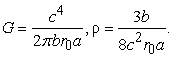These functions of the scale factor a are inserted into eq. (11). It appears that the ρ-term and the p-term cancel each other. However, to explain expansion we need an extra pressure. As a solution a new parameter β was introduced in order to account for the action provided by the continuous addition of new matter. This is a more fruitful way to explain expansion than to invent a new type of cosmological constant.

The final acceleration equation takes the form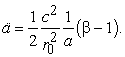(12)

For β = 1, the acceleration becomes zero, the unstable case, which bothered Einstein and was overthrown by Hubble.

As a first step in finding the first derivative of a we write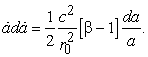(13)

By integration we obtain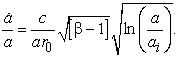(14)

Here ai = ri/r0 = 1,2682·10-42. Eq. (14) is the Hubble parameter h. The ordinary H number is obtained by multiplying with kh =3,08567·1022 m/Mpc.

The universe’s age is obtained by integration of eq. (14). The mathematics involves error functions, cf. 6, the result is of the form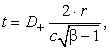(15)

where D(√ln(a/ai)) is the Dawson integral function. Notice that ar0 = r has been substituted into the equation.

In order to determine the parameter β we need to know the Hubble parameter Ho of the present time. In Ref. 6 H0 was determined by calibrating the age-to-proper distance diagram with the Planck satellite results. H0 was found to be 68,24 km/(sMpc) (similar estimates ware also presented in Ref. 16). From eq. (14) β was then determined: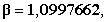which is slightly greater than 1 indicating the expansion.

The time needed for the universe to reach its present size appears to be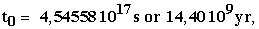which is 3,9 % greater than recently estimated.

The acceleration of the present universe expansion is obtained from eq. (12)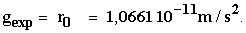g was chosen as a common symbol for acceleration to avoid a mix-up with the scale factor a.

Eq. (12) is exceptionally interesting as it indicates that the acceleration is an intrinsic characteristic of the universe. For any arbitrary comoving distance d = ar0 the acceleration is always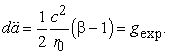(16)

In order to test the validity of the CBU theory we compare the temporal development of the Hubble parameter of different approaches, Figure 2. The CBU curve was determined according to eqs (14) and (15). Based on typical ΛCDM parameters John Rennie calculated the function H(t), 15. The lower curve is based on NASA satellite data, 17. Considering the logarithmic Hubble scale the agreement between the different approaches is acceptably good. ΛCDM is a statistically fitted model, whereas CBU is a mathematical theory, firmly anchored in physics.

• Figure 2. Comparison of the temporal development of the Hubble parameter

### 5. Schrödinger Equation of the Initial Event

The classical time-independent Schrödinger equation is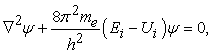(17)

where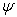is the quantum-mechanical wave function, Ei is the ground state energy, Ui = 4πbri2 is the potential energy. The spherically symmetric form of eq. (17) is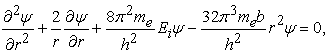(18)

where r is the curvature radius and b the energy constant of eq. (9).

Let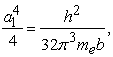(19)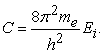(20)

Now the Schrödinger equation takes the form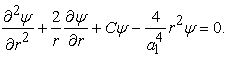(21)

The differential equation is of the Sturm-Liouville type, for which we have the solution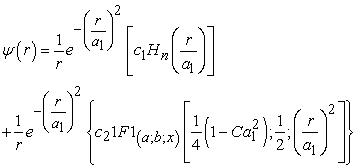(22)

where Hn is the Hermite polynomial function and 1F1(a;b;x) is the Kummer confluent hypergeometric function. c1 and c2 are constants of integration.

We have a special interest in the constant a1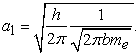(23)

By substituting b from eq. (9) into the square root denominator we have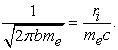(24)

Further, by substituting Gi from eq. (3) we end up with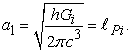(25)

By definition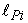is the Planck length of the virgin universe. In the CBU theory the Planck length is dependent on the curvature radius r. The numerical value is= 1,435164·10-14 m, i.e. 26,907 times the initial radius ri.

This is a ground-breaking result, which indicates that the hypotheses at the roots of the CBU theory are highly credible.

In order to show the connection between the birth of the universe and a quantum fluctuation we form the ratio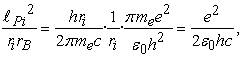(26)

where rB is the Bohr radius of the hydrogen atom. The last term equals the fine structure constant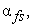we have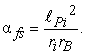(27)

The equation is a manifestation of the link between gravity and quantum mechanics. It underlines the significance of the curvature radius ri of the virgin universe.

Substituting G from eq (10) into eq. (25) we obtain a general expression for the Planck length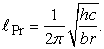(28)

A check with the present r = r0 = 4,2055083·1026 m and b = 0,4580139·1017 J/m2 results in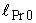= 1,616255·10-35 m, i.e. the official Planck length.

In analogy with the hydrogen atom the ground state energy Ei is postulated to be of the form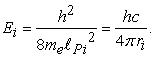(29)

The general expression of the instantaneous ground state energy takes the form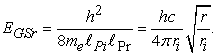(30)

It is logical to assume that EGSr represents the energy of the quantum foam, as first suggested by John Archibald Wheeler, 19, i.e. the instantaneous value of the virtual vacuum energy.

### 6. Generalized Uncertainty

By definition the fluctuation of a positron-electron pair should match the Heisenberg uncertainty principle.

Let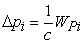(uncertainty of momentum) and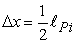(uncertainty of location), then we have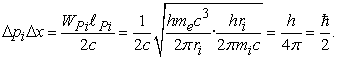(31)

This is the expected result.

However, the extremely rapid change of momentum of the expanding universe requires a modification of the uncertainty principle, cf. Ronald J. Adler, 20. Instead of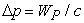we introduce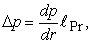(32)

where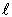Pr is the Planck length at any r. The momentum change with respect to r is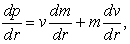(33)

where v = r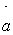= the velocity of the expansion. From eq. (14) we have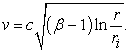(34)

Further by using the relation m = 4πbr2/c2 we determine the momentum change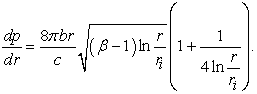(35)

The generalized uncertainty now takes the form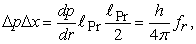(36)

where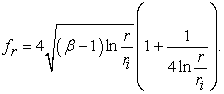(37)

### 7. The Planck Energy

The Planck energy is of crucial importance for the determination of the number of positron-electron pairs becoming real out of the virtual foam particles. By definition we have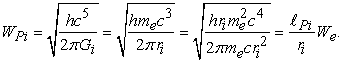(38)

The energy of the positron-electron pair is 2We. A pair is a fraction of the Planck energy according to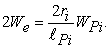(39)

The equation tells how many fluctuations occur during the related Planck time tPi. The ratio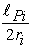= 13,4535 is responsible for the creation of new matter and accordingly the expansion of the universe.

For arbitrary values of the radius r the Planck energy takes the form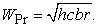(40)

A check with the present r = r0 = 4,2055083·1026 m and b = 0,4580139·1017 J/m2 results in WPr0 = 1,9560815·109 J = mPo·c2 = 2,17643·10-8c2, i.e. in complete conformity with the official Planck mass.

It can easily be shown that the instantaneous ground state vacuum energy EGSr is directly proportional to WPr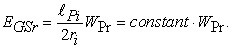(41)

We now have all necessary ingredients to show that the fluctuations of positron-electron pairs during a period of the Planck time’s length produce a continuous inflow of new matter in a manner that exactly predicts the present expansion of the universe.

### 8. The Proof

The time derivative of incoming matter is set to equal the Planck energy divided by the Planck time, tPr =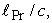and further multiplied by fr to observe the generalized uncertainty: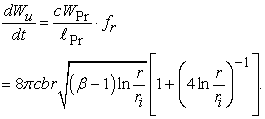(42)

The bracket to the right is a correction factor due to the fact that the time derivative of the scale factor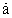is an approximation. The influence of the correction factor is very small, which indicates the validity of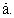It also indicates that  is nearly a constant.

In order to prove that the total amount of positive energy is built up according to eq. (42), we solve the time integral: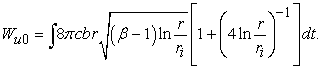(43)

The curve fit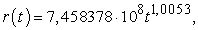(44)

obeys eq. (15) with a very high accuracy.

The numerical integration was performed with WolframAlpha, Figure 3. The result, 1,01822·1071 J, is almost identical with the original value of eq. (8):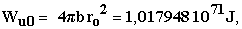the grand total of the energy of the universe. The lower integration limit t =10-24 s is very close to the initial moment. The influence of the correction factor is insignificant.

• Figure 3. Screenshot of the numerical solution to the integral of eq. (43)

Remark. The CBU theory does not require dark energy, because expansion is due to the inflow of matter. However, new matter stems from the instantaneous virtual ground state energy. According to the ΛCDM model this energy accumulates into the dark energy WDE.

From eq. (41) we have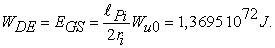(45)

### 9. Comparison ΛCDM versus CBU

Equation (45) implies thatPi/2ri equals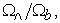where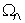and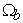represent the ΛCDM parameters of dark and baryonic energy respectively. However, there is a discrepancy in the ratio of omegas. From the familiar black body equations (cf. Wikipedia: Photon gas) we deduce that the present CMB photon energy for T0= 2,7255 K, is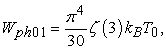(46)

where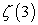= 1,2020569 is the Riemann zeta-function and kB the Boltzmann constant. On the other hand, the photon energy is Wph02 = hf0. f0 (160,23·109 Hz) and T0 are related to the peak value of the Planck black body distribution such that hf0/kBT0 = 2,821439. We form the ratio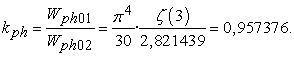(47)

When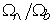is multiplied with the discrepancy factor kph we should obtainPi/2ri (13,45). From recent satellite data, 15 and 18, we have typically= 0,689,= 0,0490. The ratio is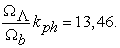This implies that the baryonic content is slightly larger than the ΛCDM model presumes.

In Ref. 14 the critical density was estimated to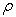cr = 8,54·10-27 kg/m3. Here the Hubble parameter is slightly higher increasing the density to 8,79·10-27 kg/m3. The density of matter (and a very small portion of radiation) isb =cr/kph = 0,4499·10-27 kg/m3. The CBU value is 0,454·10-27 kg/m3.

The dark energy density (divided by c2) of ΛCDM is=cr =6,06·10-27 kg/m3. The corresponding CBU value, eq. (45), would be 6,11·10-27 kg/m3.

### 10. Conclusions

There are several strong arguments in favour of the CBU theory. It has been shown that the positive energy of the universe follows a squared radius law, which, starting with a positron-electron vacuum fluctuation, predicts the present content of baryonic and electromagnetic energy to a high precision.

In an article by the present author the CBU theory led to the derivation of an inherent acceleration (1,066·10-11 m/s2) of the expanding universe. The acceleration causes a Coriolis effect, which explains the unexpected rotational pattern of the galaxies. No dark matter is required.

In the present article it is shown that quantum mechanics is firmly linked to the birth of the universe and to the continuous creation of new matter. The Einstein field equation can be returned into its original format, wherein the geometric G tensor equals the energy-momentum T tensor thereby omitting the need of a cosmological constant, 15. However, the Einstein equation must be reformulated in order to take into account the size dependence of the gravitational parameter G. A corrected formulation could shed new light on the relationship between gravitation and quantum mechanics.

Nucleosynthesis is an important argument in support of the standard model. According to the theory protons and neutrons are formed when the temperature drops below 1012 K and quarks are not allowed to be free. There are, however, several obstacles to a general acceptance of the standard model. The problems have been circumvented by the introduction of still unrecognized physical concepts.

According to the CBU theory matter is created spontaneously throughout space. So far, the transmutation of electrons and positrons into fermions is an unanswered question. What is the impact of the black holes in the galaxy centres? Does the gravitational pull make positron-electron clouds dive into the ovens of stars? Or are the clouds able to implode and create the nucleus of new stars? We know that matter has a tendency to cluster around black holes, that’s why we have stars and galaxies. A change of paradigm could bring new answers to these questions.

### References

  Hoyle, F., A new model for the expanding universe, Mon. Not. Roy. Ast. Soc., 108, pp. 372-382, 1948. In article View Article  Bondi, H., Gold, T., The steady-state theory of the expanding universe, Mon. Not. Roy. Ast. Soc., 108, pp. 252-270, 1948. In article View Article  Tryon, E. P., Is the universe a vacuum fluctuation, Nature, 247, pp. 396-397, 1973. In article View Article  Dirac, P., Cosmological models and the Large Number hypothesis, Proc. R. Soc. Lond. A., 338, pp. 439-446, 1974. In article View Article  Guth, A., The Inflationary Universe: The quest for a new theory of cosmic origins, Perseus Books, 1997. In article View Article  Eriksson, J.-T., A modified model of the universe shows how acceleration changes galaxy dynamics, International Journal of Physics, Vol 6, No. 2, pp. 38-46, 2018. In article  Eriksson, J.-T., The momentum of new matter replaces dark energy and explains the expansion of the universe, International Journal of Physics, Vol. 6, no. 5, pp. 161-165, 2018. In article View Article  Eriksson, J.-T., A combined cosmological and gravitational redshift supports Electron-positron annihilation as the most likely energy source of the CMB, International Journal of Physics, Vol. 7, no. 1, pp.16-20, 2019. In article View Article  McBryan, B., Living in a low-density black hole, arXiv: 1312.0340v1, 2 Dec 2013. In article  Einstein, A., Kosmologische Betrachtungen zur allgemeinen Relativitätstheorie. Sitzungsberichte der Preussischen Akad. d. Wissenschaften, 1917. In article  Sciama, D. W., On the origin of inertia, Monthly Notices of the Royal Astronomical Society, 113, 34-42, 1952. In article View Article  Brans, C., Dicke, R. H., Mach's principle and a relativistic theory of gravitation, Physical Review, 124, 3, pp. 925-935, 1961. In article View Article  Fahr, H.-J., Sokaliwska, M., Remaining problems in interpretation of the Cosmic Microwave Background, Physics Research International, Vol. 2015, Article ID 503106, 2015. In article View Article  Tanabashi, M. et al., Astrophysical constants and parameters, Phys.Rev. D 98, 2018 (2019 update). In article  Einstein, A., Die Grundlage der allgemeine Relativitätstheorie. Annalen der Physik, 49, 769-822, 1916. In article View Article  Planck Collaboration: Aghanin, N., et al, Planck 2018 results. Cosmological parameters, arXiv:1807.06209v2, 20 Sep 2019. In article  Rennie, J., StackExchange, Sept 2014. In article  Nemiroff, R., Bonnell, J., Astronomy picture of the day, April 8, 2013. In article  Wheeler, J. A., Geons, Physical Review 97 (2): 511-536, 1955. In article View Article  Adler, R. J., Six easy roads to the Planck scale, Am. J. Phys., 78, pp. 925-932, 2010. In article View ArticleThis work is licensed under a Creative Commons Attribution 4.0 International License. To view a copy of this license, visit http://creativecommons.org/licenses/by/4.0/